# Humming Bird - HMO PDF Sample Papers for Class 2

Resources:

Class 2 sample paper & practice questions for Humming Bird Mathematics Olympiad (HMO) level 1 are given below. Syllabus for level 1 is also mentioned for these exams. You can refer these sample paper & quiz for preparing for the exam.Q.1 Q.2 Q.3 Q.4 Q.5 Q.6 Q.7 Q.8 Q.9 Q.10
 Q.1 Find the missing weight to balance the scale.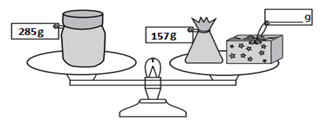a) 138 g b) 128 g c) 118 g d) 12.8 g
 Q.2 The cost of one red box is Rs. 100, one blue box is Rs. 50, and one green box is Rs. 20. Find the total cost of the boxes given below.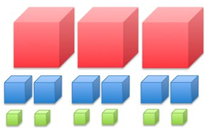a) Rs. 580 b) Rs. 640 c) Rs. 720 d) Rs. 880
 Q.3 Find the missing weight to balance the scale.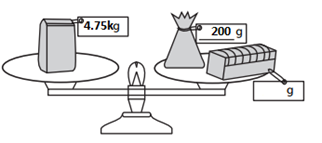a) 3550 kg b) 455 kg c) 4.55 kg d) 4550 kg
 Q.4 Find the middle of the ruler.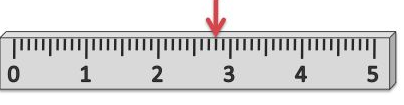a) Exactly below the red arrow b) To the left of the red arrow c) To the right of the red arrow d) Can not find
 Q.5 Three items having weight 86 kg, 79 kg and 65 kg respectively. Find their total weight. a) 210 kg b) 240 kg c) 220 kg d) 230 kg
 Q.6 In how many hours, time shown in clock 1 will reach to the time shown in clock 2?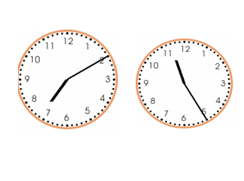a) 4 hour 50 minutes b) 5 hour 30 minutes c) 7 hour 45 minutes d) 4 hour 15 minutes
 Q.7 Shyam gave Rs. 500 to a shopkeeper and bought three items of Rs. 217, Rs. 85, and Rs. 69 respectively. How much money did he get in return from the shopkeeper? a) Rs. 371 b) Rs. 189 c) Rs. 139 d) Rs. 129
 Q.8 Which circle contains the smallest number of parts? a)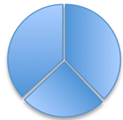b)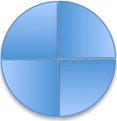c)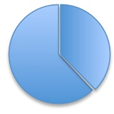d)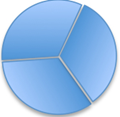Q.9 Which of the following image represents semi-circle? a)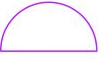b)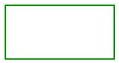c)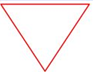d)Q.10 Which three numbers among the following options have a sum of 789? a) 70 + 80 + 9 b) 700 + 800 + 9 c) 699 + 79 + 11 d) 700 + 89 + 9Sample PDF of Humming Bird - Humming Bird Mathematics Olympiad (HMO) PDF Sample Papers for Class 2: